# 1.0 introduction to biomechanics

24 de Jul de 2019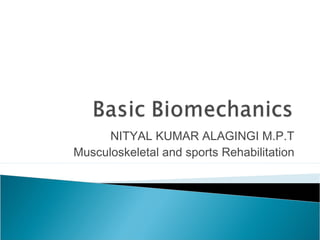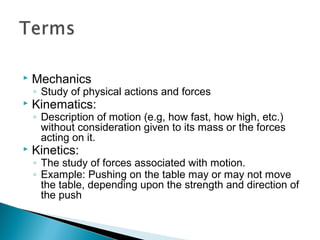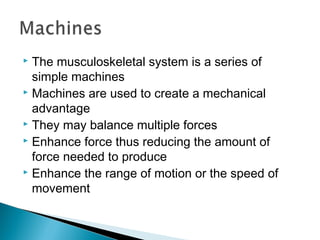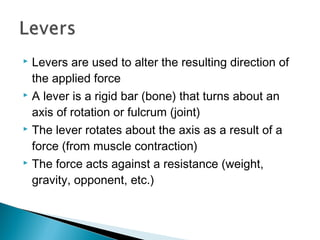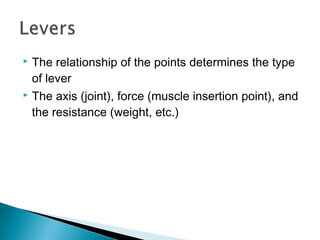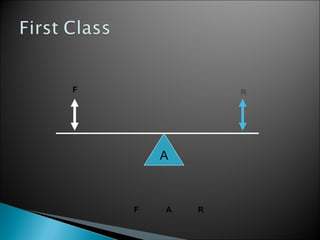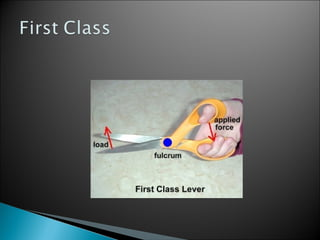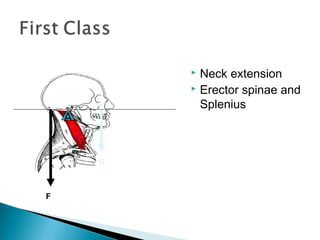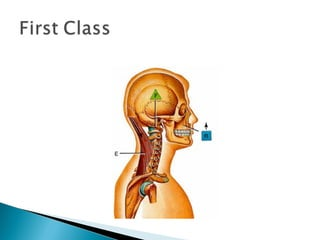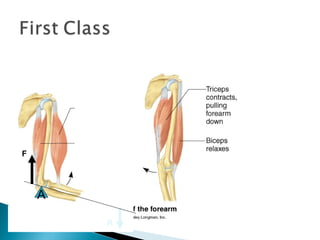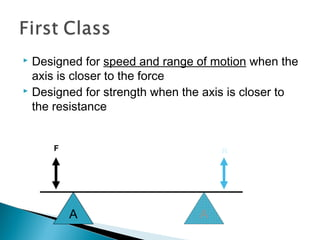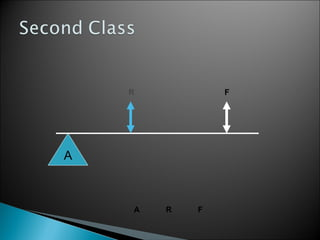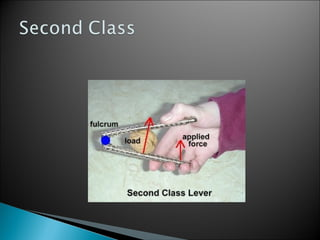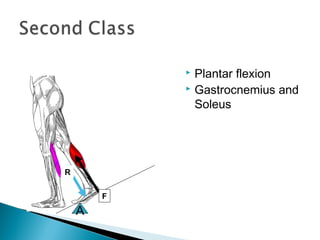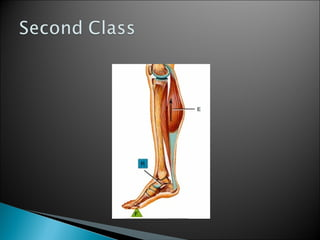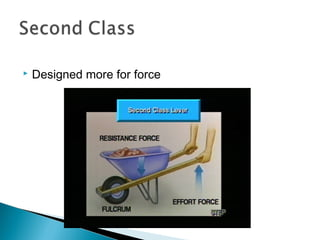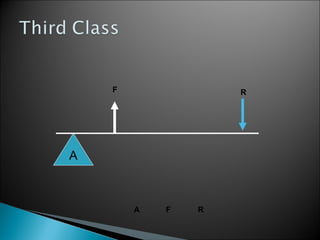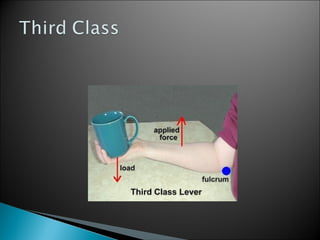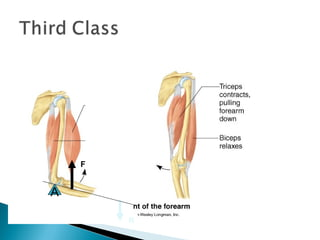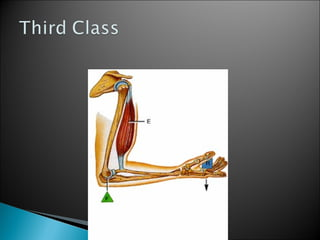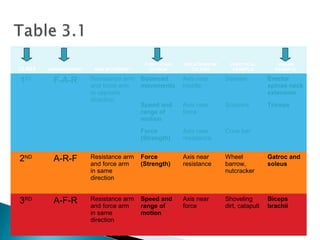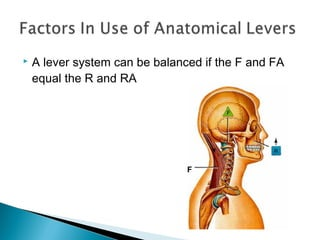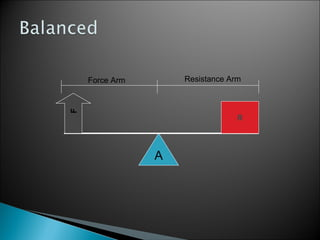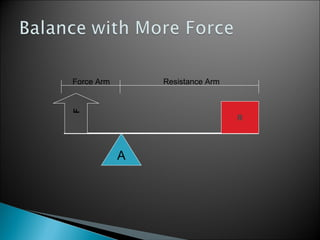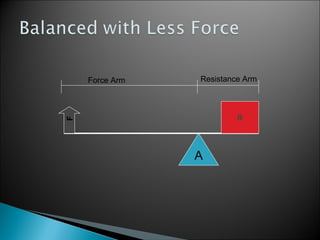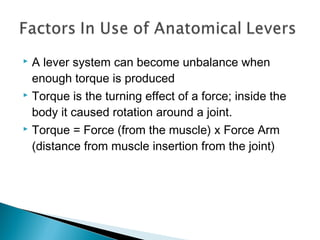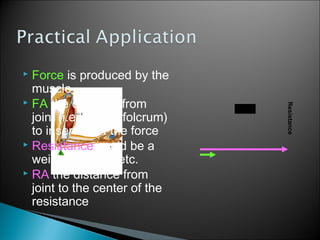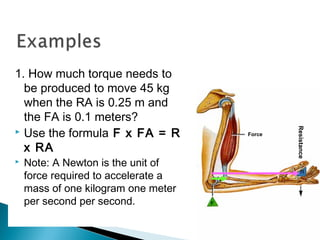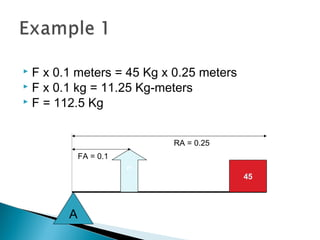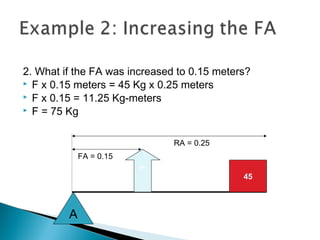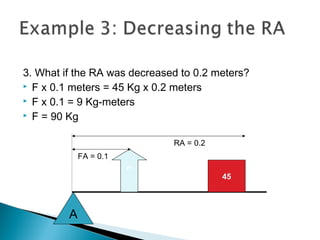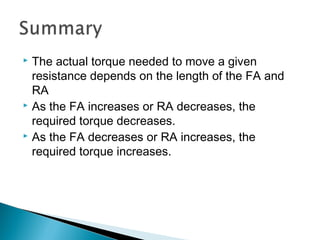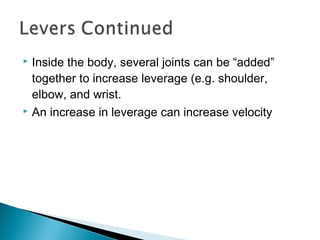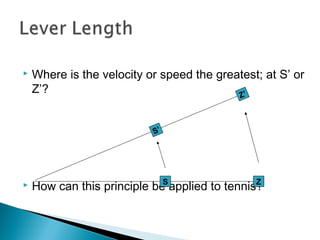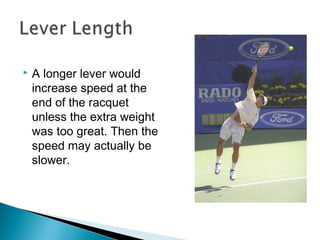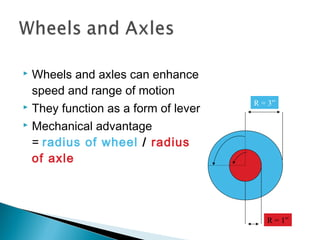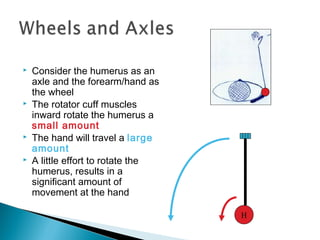1 de 38

### 1.0 introduction to biomechanics

• 1. NITYAL KUMAR ALAGINGI M.P.T Musculoskeletal and sports Rehabilitation
• 2.  Mechanics ◦ Study of physical actions and forces  Kinematics: ◦ Description of motion (e.g, how fast, how high, etc.) without consideration given to its mass or the forces acting on it.  Kinetics: ◦ The study of forces associated with motion. ◦ Example: Pushing on the table may or may not move the table, depending upon the strength and direction of the push
• 3.  The musculoskeletal system is a series of simple machines  Machines are used to create a mechanical advantage  They may balance multiple forces  Enhance force thus reducing the amount of force needed to produce  Enhance the range of motion or the speed of movement
• 4.  Levers are used to alter the resulting direction of the applied force  A lever is a rigid bar (bone) that turns about an axis of rotation or fulcrum (joint)  The lever rotates about the axis as a result of a force (from muscle contraction)  The force acts against a resistance (weight, gravity, opponent, etc.)
• 5.  The relationship of the points determines the type of lever  The axis (joint), force (muscle insertion point), and the resistance (weight, etc.)
• 8.  Neck extension  Erector spinae and Splenius A F R
• 10.  Elbow extension  Triceps A F R
• 11.  Designed for speed and range of motion when the axis is closer to the force  Designed for strength when the axis is closer to the resistance A F R A
• 14.  Plantar flexion  Gastrocnemius and Soleus A F R
• 16.  Designed more for force
• 19.  Elbow flexion  Biceps brachii and Brachialis A F R
• 21. CLASS ARRANGEMENT ARM MOVEMENT FUNCTIONAL DESIGN RELATIONSHIP TO AXIS PRACTICAL EXAMPLE HUMAN EXAMPLE 1ST F-A-R Resistance arm and force arm in opposite direction Balanced movements Axis near middle Seesaw Erector spinae neck extension Speed and range of motion Axis near force Scissors Triceps Force (Strength) Axis near resistance Crow bar 2ND A-R-F Resistance arm and force arm in same direction Force (Strength) Axis near resistance Wheel barrow, nutcracker Gatroc and soleus 3RD A-F-R Resistance arm and force arm in same direction Speed and range of motion Axis near force Shoveling dirt, catapult Biceps brachii
• 22.  A lever system can be balanced if the F and FA equal the R and RA F
• 26.  A lever system can become unbalance when enough torque is produced  Torque is the turning effect of a force; inside the body it caused rotation around a joint.  Torque = Force (from the muscle) x Force Arm (distance from muscle insertion from the joint)
• 27.  Force is produced by the muscle  FA the distance from joint (i.e. axis or folcrum) to insertion of the force  Resistance could be a weight, gravity, etc.  RA the distance from joint to the center of the resistance Force Resistance
• 28. 1. How much torque needs to be produced to move 45 kg when the RA is 0.25 m and the FA is 0.1 meters?  Use the formula F x FA = R x RA  Note: A Newton is the unit of force required to accelerate a mass of one kilogram one meter per second per second. Force Resistance
• 29.  F x 0.1 meters = 45 Kg x 0.25 meters  F x 0.1 kg = 11.25 Kg-meters  F = 112.5 Kg A 45 ? FA = 0.1 RA = 0.25
• 30. 2. What if the FA was increased to 0.15 meters?  F x 0.15 meters = 45 Kg x 0.25 meters  F x 0.15 = 11.25 Kg-meters  F = 75 Kg A 45 ? FA = 0.15 RA = 0.25
• 31. 3. What if the RA was decreased to 0.2 meters?  F x 0.1 meters = 45 Kg x 0.2 meters  F x 0.1 = 9 Kg-meters  F = 90 Kg A 45 ? FA = 0.1 RA = 0.2
• 32.  The actual torque needed to move a given resistance depends on the length of the FA and RA  As the FA increases or RA decreases, the required torque decreases.  As the FA decreases or RA increases, the required torque increases.
• 33.  Inside the body, several joints can be “added” together to increase leverage (e.g. shoulder, elbow, and wrist.  An increase in leverage can increase velocity
• 34.  Where is the velocity or speed the greatest; at S’ or Z’?  How can this principle be applied to tennis? S’ Z’ S Z
• 35.  A longer lever would increase speed at the end of the racquet unless the extra weight was too great. Then the speed may actually be slower.
• 36.  Wheels and axles can enhance speed and range of motion  They function as a form of lever  Mechanical advantage = radius of wheel / radius of axle R = 3” R = 1”
• 37.  Consider the humerus as an axle and the forearm/hand as the wheel  The rotator cuff muscles inward rotate the humerus a small amount  The hand will travel a large amount  A little effort to rotate the humerus, results in a significant amount of movement at the hand H